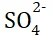# Liquid Junction Potential## Liquid Junction Potential:

When a cell is formed by the combination of two half cells or electrodes having two solutions either of different electrolytes (electrochemical cell) or of the same electrolyte but at different concentrations (concentration cell), a liquid junction is formed where these two solutions meet. At the junction of two liquids of solutions, an electric potential is developed due to the differential rates of migrations of ions across the boundary between the two solutions. This is known as liquid junction potential (EJ).

This potential is in addition to the two electrode potentials in a cell and is thus, included or added to the EMF of the cell. Therefore, we have

Where ‘ER‘ and ‘EL‘ are single electrode potential (reduction) of the two half-cells combing and ‘EJ‘ is the liquid junction potential.

### Origin of Liquid Junction Potential:

Case I (In electrochemical cells): Let us consider a Daniel cell containing solutions of ZnSO4 and CuSO4 of equal molality ‘m’, i.e., Zn | ZnSO4 (m) : CuSO4 (m) | Cu.

This cell is schematically represented in the above figure. At the Junction between two solutions, there is diffusion of ions from one solution to the other.

We have equal concentrations ofions in both solutions. Now Cu2+ ions have a bit greater mobility than Zn2+ ions in aqueous solutions. Since the rate or speed of diffusion of an ion is in a way proportional to the mobility of the ion, Cu2+ ions diffuse faster through the liquid junction to the side of ZnSO4 solution than Zn2+ ions diffuse into CuSO4 solution. This leads to a small excess of positive charge on the ZnSO4 side and a small excess negative charge on the CuSO4 side increasing the rate of diffusion of Zn2+ ions into CuSO4 solution till an equilibrium state is reached when rates of diffusion of two ions across the boundary become equal. In this state, there is a small but net positive charge on one side and a small but net negative charge on the other side of the boundary. This produces a very small potential difference between the ZnSO4 solution and CuSO4 solution at the liquid junction (liquid junction potential) which also contributes to the measured EMF of the cell.

Case II (In concentration cells): In a cell of the type, M | MA (m1) : MA (m2) | M, there are two solutions of the same binary electrolyte, MA, but at different concentrations. (With m2 > m1) in contact with each other. Here both the ions of the electrolyte diffuse from the more concentrated solution side to the less concentrated solution side.

(a) If the speed of the cation (M+) is more than that of the anion (A) of an electrolyte, the cation will diffuse a head of the anion into a more dilute solution across the boundary between the solutions. Therefore, a slight positive charge on the less concentration (or more dilute) side and a slight negative charge on the more concentration side of the junction is produced. Such is the case in cells.

Pt | H2 (1 atm) | HCl (a1) | HCl (a2) | H2 (1 atm) | Pt, a2 > a1, where the speed of H+ is more than that of Cl.

(b) If the speed of the anion (A) is more than that of the cation (M+), the anion will diffuse faster than the cation from the more concentration side to the more dilute side so that a slight negative charge is produced on the more dilute side of the junction. Such a process occurs in a cell, Cu | CuSO4 (m1) | CuSO4 (m2) | Cu, m2 > m1, where the migration speed ofion is more than that of the Cu2+ ion.

Thus, we find that in both cases (a) and (b), the more dilute side acquires the charge of the faster-moving ion as a result of the difference in the migration velocities of two ions diffusing across the boundary. In other words, there is separation of charges or an electrical double layer is produced at the junction. This gives rise to liquid junction potential.

(c) If the speeds of two diffusing ions of the binary electrolyte are equal, no liquid junction potential will arise.